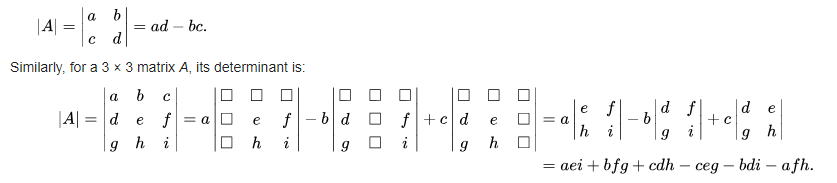﻿ NumPy: Compute the determinant of a given square array - w3resource# NumPy: Compute the determinant of a given square array

## NumPy: Linear Algebra Exercise-4 with Solution

Write a NumPy program to compute the determinant of a given square array.

From Wikipedia: In linear algebra, the determinant is a value that can be computed from the elements of a square matrix. The determinant of a matrix A is denoted det(A), det A, or |A|. Geometrically, it can be viewed as the scaling factor of the linear transformation described by the matrix.

In the case of a 2 × 2 matrix the determinant may be defined as:Sample Solution :

Python Code :

``````import numpy as np
from numpy import linalg as LA
a = np.array([[1, 0], [1, 2]])
print("Original 2-d array")
print(a)
print("Determinant of the said 2-D array:")
print(np.linalg.det(a))
``````

Sample Output:

```Original 2-d array
[[1 0]
[1 2]]
Determinant of the said 2-D array:
2.0
```

Python Code Editor:

Have another way to solve this solution? Contribute your code (and comments) through Disqus.

What is the difficulty level of this exercise?

Test your Python skills with w3resource's quiz

﻿

## Python: Tips of the Day

```print(2_000_000)
```2000000